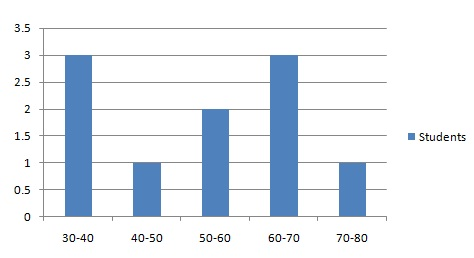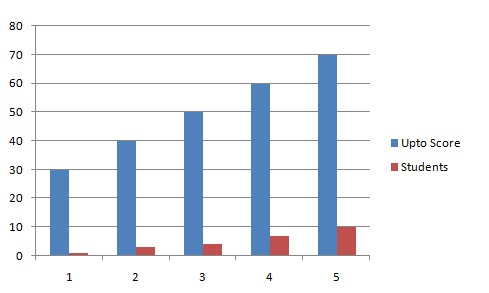# Statistics - Cumulative plots

A cumulative plot is a way to draw cumulative information graphically. It displays the number / percentages, or proportion of observations that are less than or equal to particular value.

## Problem Statement:

Draw the frequency and comulative frequency plots of 10 student test scores based on following data.

Sr. No.Roll No.Test Score
110030
210140
310235
410350
510460
610565
710535
810555
910565
1010570

## Solution:

For the frequency chart, compute the frequecies as shown below. This table show the no. of students scoring in given ranges.

Sr. No.FrequencyStudents
130-403
240-501
350-602
460-703
470-801

Following is the required frequency plotFor the comulative frequency chart, compute the frequecies as shown below. This table show the no. of students scoring upto given marks(including).

Sr. No.Upto ScoreStudents
1301
2403
3504
4607
57010

Following is the required frequency plot